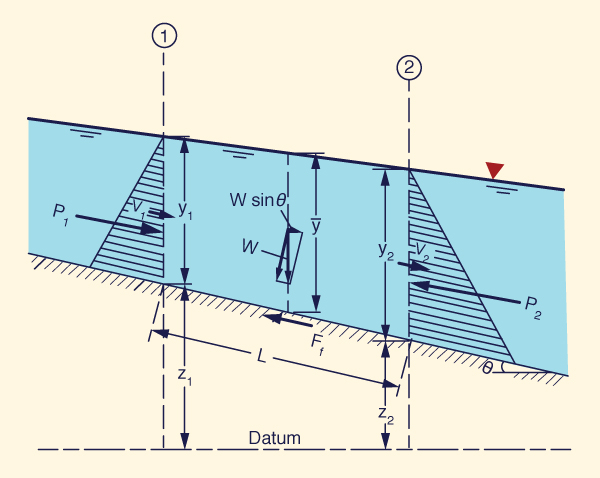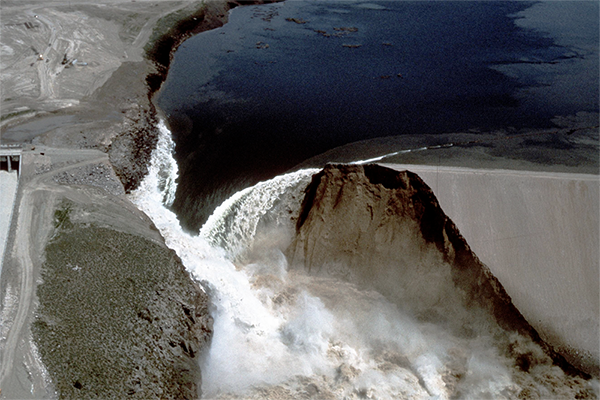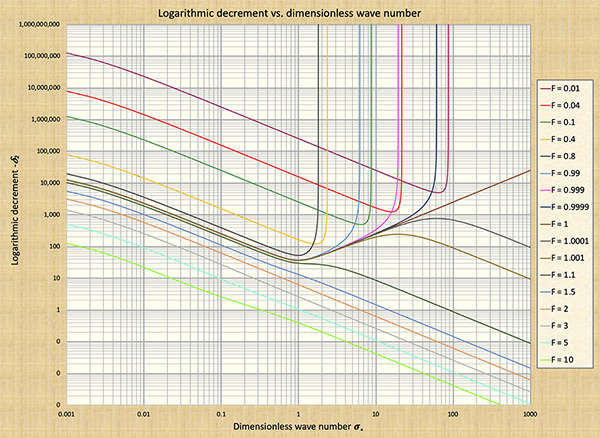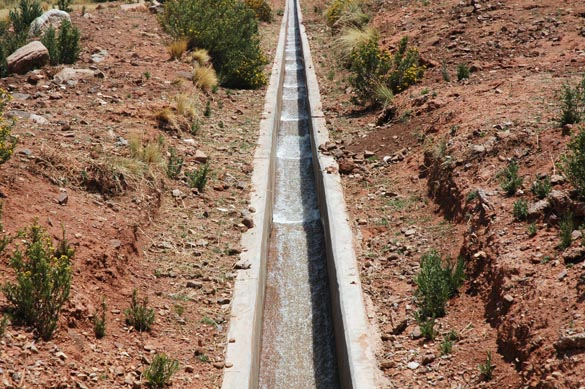The S-curve explained Victor M. Ponce and Janaina Da Silva27 October 2019

 Abstract. The theory of shallow wave propagation of Ponce and Simons (1977) is reviewed and clarified, with aim to showcase its importance in understanding the mechanics of unsteady open-channel flow. Four forces are identified at the outset: gravity, friction, pressure gradient, and inertia. These forces give rise to several practical wave combinations, enabling the definition of the following types of shallow waves: (1) kinematic, (1a) diffusion, (2) mixed kinematic-dynamic, and (3) dynamic waves. The propagation characteristic of these wave types, including celerity and attenuation functions, are described and explained in detail. Elucidation of the theory should make possible increased understanding and lead to better modeling practice.

1.  INTRODUCTION

The dichotomy between kinematic and dynamic waves and its relation to scale has defied complete understanding in the past. The matter has been elucidated by Ponce and Simons (1977), who identified the appropriate dimensionless wavenumber and plotted dimensionless relative wave celerities across the spectrum of dimensionless wavenumbers. In addition, they clarified the propagation characteristics of various types of shallow water waves, including their celerity and attenuation properties. Herein, we review the Ponce and Simons theory and explain its significance. The aim is to showcase the importance of wave propagation in hydraulic engineering modeling practice.

2.  THE THEORY EXPLAINED

Shallow wave propagation in open-channel flow is governed by the equations of water continuity and motion, generally referred to as the Saint Venant equations (Ponce, 2014). These are a set of two partial differential equations for which there is no complete analytical solution. This theoretical difficulty was circumvented by Ponce and Simons (1977), who linearized the governing equations and applied the tools of linear stability to formulate a characteristic algebraic equation which retained the essence of the solution.

The proper definition of dimensionless wavenumber allowed the calculation of celerity and attenuation functions across the entire spatial domain. The results described wave propagation for several types of shallow waves, throwing much needed light on the wave phenomena.

3.  FORCES AND WAVE TYPES

There are four forces in the analysis of unsteady open-channel flow (Fig. 1): (1) gravitational (gravity) (W sinθ ); (2) bottom friction (friction) (Ff ), (3) pressure gradient (P2 - P1)/L, and (4) inertial (inertia) (not shown).Fig. 1  Forces acting on a control volume (inertia force not shown).

The following types of shallow water waves are in general use: (1) kinematic, (1a) diffusion, (2) mixed kinematic-dynamic, and (3) dynamic. Kinematic waves are governed solely by gravity and friction (Fig. 2); diffusion waves by gravity, friction and the pressure gradient; mixed kinematic-dynamic waves by all four forces, namely gravity, friction, the pressure gradient, and inertia (Fig. 3); and dynamic waves solely by the pressure gradient and inertia (Table 1).

 Table 1.  Types of shallow water waves. No.(1) Wave type(Name used here)(2) Terms of the equation of motion included Common name(7) Applications(8) Gravity(3) Friction(4) Pressure gradient(5) Inertia(6) 1 Kinematic ✓ ✓ Kinematic Overland flow,flood routing 1a Diffusion ✓ ✓ ✓ Diffusion Flood routing 2 Mixed kinematic-dynamic ✓ ✓ ✓ ✓ Dynamic Dam-breach flood routing,flood routing 3 Dynamic ✓ ✓ Gravity Laboratory flumes,small canal operation

At the outset, it is necessary to point out that there exists currently a semantic confusion regarding what to call the different types of waves. What we call here mixed kinematic-dynamic waves have been generally referred to in the literature as "dynamic," following the work of Fread (1973). On the other hand, the classical dynamic waves, i.e., the dynamic waves of Lagrange (1788), have been often referred to as "gravity" waves. In this work, we follow the naming indicated in Column 2 of Table 1, in an effort to settle the semantic confusion.Fig. 2  A kinematic wave, featuring a flood wave period of one year: Upper Paraguay river,
at Porto Murtinho, Mato Grosso do Sul, Brazil.

The term "kinematic wave" was introduced by Lighthill and Whitham in their seminal paper, purportedly to contrast the then well established dynamic waves, which transport energy, to waves that transport mass. We quote here directly from Lighthill and Whitham (1955):

 "... the [kinematic] wave property follows from the equation of continuity alone. Accordingly, we suggest that the[se] waves be described as "kinematic." The classical wave motions would in contrast be described as "dynamic" waves, depending as they do on Newton's second law of motion [inertia]."

Classical dynamic waves transport only energy; therefore, it follows that mixed kinematic-dynamic waves transport both mass and energy. In the resolution of this dichotomy may lie the strong attenuating (dissipating) tendency of mixed kinematic-dynamic waves.Fig. 3  Failure of Teton Dam, on the Teton river, Idaho, on June 5, 1976,
releasing a mixed kinematic-dynamic wave.

4.  PONCE AND SIMONS' FINDINGS

Ponce and Simons plotted dimensionless relative wave celerities across the spectrum of dimensionless wavenumbers and specialized their findings into each one of the four wave types described in Table 1. Crucial to this effort was the identification of the dimensionless wavenumber σ* :

 2 π σ*  =    ______   Lo                 L
(1)

in which Lo = characteristic reach length, the length of channel in which the steady uniform flow drops a head equal to its depth (Lighthill and Whitham, 1955):

 do Lo  =    ______                 So
(2)

in which do = steady uniform flow depth, and So = channel bed slope.

Figure 4 shows dimensionless relative wave celerities across the dimensionless wavenumber spectrum. Given c = wave celerity, the relative wave celerity cr is:

 cr  =  c - uo
(3)

in which u o = steady uniform flow velocity. The dimensionless relative wave celerity cr*  is:

 c - uo cr*  =    _______                   uo
(4)

The range of dimensionless wavenumbers shown in Fig. 4 spans six orders of magnitude, encompassing from the longest waves (kinematic, of small σ*), to the shortest waves (dynamic, of large σ*). Herein, we refer to Fig. 4 as the S-curve. All four wave types (kinematic, diffusion, mixed kinematic-dynamic, and dynamic) are contained in the S-curve.Fig. 4  Dimensionless relative wave celerity vs dimensionless wavenumber in open-channel flow
(Ponce and Simons, 1977).

5.  KINEMATIC WAVES

Kinematic waves are governed solely by gravity and friction. Their celerity is constant and equal to the kinematic wave celerity, or Seddon celerity, and they do not attenuate (with some exceptions for nonlinear waves). The Seddon celerity is (Seddon, 1900):

 1           dQ ck =    _____   ______              T            dy
(5)

in which T = channel top width, Q = discharge, and y = flow depth. The Seddon, or kinematic wave celerity, is alternatively expressed as follows (Ponce, 2014):

 ck  =   β u
(6)

in which β = exponent of the discharge-flow area rating:

 Q  =   α A β
(7)

The relative kinematic wave celerity, or kinematic wave celerity relative to the flow velocity, is:

 crk   =  (β - 1) u
(8)

The dimensionless relative kinematic wave celerity is:

 cdrk  =   β - 1
(9)

The value of β is a function of channel friction and cross-sectional shape. For Chezy friction in a hydraulically wide channel: β = 1.5. In this case:

 cdrk  =   0.5
(10)

For Froude numbers in the stable regime, F ≤ 2, which corresponds to Vedernikov numbers V ≤ 1 (Ponce, 1991), Fig. 4 shows the range of dimensionless wavenumbers for which kinematic waves prevail: 0.001 ≤ σ* ≤ 0.1. For Froude and Vedernikov numbers in the unstable regime, F > 2, or V > 1, the range is somewhat reduced, as shown to the left of Fig. 4.Fig. 4  Dimensionless relative wave celerity vs dimensionless wavenumber in open-channel flow
(Ponce and Simons, 1977).

In the Ponce and Simons theory, wave attenuation is a function of the change in relative dimensionless wave celerity with change in dimensionless wave number. In other words, attenuation is caused by the change in celerity with wave size. For the case of (dcr* /dσ*) = 0, there can be no wave attenuation; therefore, kinematic waves do not attenuate.

The above conclusion, while strong, is strictly valid only for the linear theory in hydraulically wide channels. In the general nonlinear case, kinematic waves may undergo changes in shape, either steepening or flattening, depending on the cross-sectional shape (Ponce and Windingland, 1985). In the case of steepening, this eventually leads to a kinematic shock [Fig. 5 (a)], while in the case of flattening, the situation resembles wave attenuation [Fig. 5 (b)].Fig. 5  Routing of nonlinear flood waves: (a) wave steepening; and
(b) wave attenuation (Ponce and Windingland, 1985).

In summary, kinematic waves travel with the Seddon celerity and, in general, they are not subject to attenuation. The condition of a wave being kinematic depends on the dimensionless wavenumber, with the wave becoming more kinematic as σ* decreases (See Fig. 4 for the appropriate values).

The criterion for the applicability of a kinematic wave in open-channel flow has been developed by Ponce et al. (1978). For 95% accuracy after one propagation period, the following dimensionless inequality holds:

 uo T So   ( _____ )  ≥  171                 do
(11)

in which T = wave period; So = bed slope; uo = mean velocity; and do = mean flow depth.

6.  DIFFUSION WAVES

Diffusion waves are governed by gravity, friction, and the pressure gradient. The inclusion of the pressure gradient in the formulation of a diffusion wave provides diffusion, i.e., the wave is capable of undergoing attenuation, or dissipation; therein its name, diffusion wave. The origin of the name may be traced back to the work of Lighthill and Whitham (1955), who reckoned that diffusion could be added to a kinematic wave by including the pressure-gradient term in the formulation.

In Ponce and Simons' theory, the celerity of a diffusion wave is, as an approximation, the same as that of the neighboring kinematic wave (in the dimensionless wavenumber spectrum). The difference is in the attenuation function. Unlike the kinematic wave, for which the attenuation is zero, the diffusion wave is subject to a small but finite amount of attenuation. The attenuation is a function of the dimensionless wavenumber σ* , and, as expected, increases with it.

To characterize the magnitude of the wave attenuation, Ponce and Simons used the logarithmic decrement δ, which measures the attenuation after one (sinusoidal) propagation period. For the diffusion wave, the logarithmic decrement is:

 σ* δd   =   - 2 π   ______                            3
(12)

which reduces to δd   ⇒ 0 as σ* ⇒ 0, thus coalescing into a kinematic wave.

A diffusion wave has a wider range of applicability than a kinematic wave. In cases where a kinematic wave fails, a diffusion wave may be applicable. The criterion for the applicability of a diffusion wave has been developed by Ponce et al. (1978). The criterion is based on the following dimensionless inequality:

 g T So   ( _____ ) 1/2  ≥  30                 do
(13)

in which g = gravitational acceleration.

In Figure 4, diffusion waves would fall to the right of kinematic waves, in the middle of the dimensionless wavenumber range, 0.1 ≤ σ* ≤ 10, depending on the Froude number. The lower the prevailing Froude number in the stable range (F ≤ 2), the greater the range of applicability of diffusion waves.

7.  MIXED KINEMATIC-DYNAMIC WAVES

Mixed kinematic-dynamic waves are governed by all four forces: gravity, friction, pressure gradient, and inertia. As such, a mixed kinematic-dynamic wave represents the most complete formulation of shallow-water waves in open-channel flow; however, it is not without its pitfalls. Under typical subcritical flow conditions, mixed kinematic-dynamic waves are subject to very strong attenuation, to the extent that often their existence may be placed under question. This fact was recognized by Lighthill and Whitham (1955) when they stated:

 "Under the conditions appropriate for flood waves... the dynamic (sic) waves rapidly become negligible, and it is the kinematic waves, following at a slower speed, which assume the dominant role."

Thus, the mixed kinematic-dynamic waves, lying directly on the rising limb of the S-curve (Fig. 4), are generally subject to very strong attenuation. The rate of attenuation increases with the rise on the curve, achieving a peak at the point of inflection.

These propositions were demonstrated by Ponce and Simons (1977) in their analysis of the mixed kinematic-dynamic wave model. The results are summarized in Figs. 4, 6, 7, and 8.Fig. 4  Dimensionless relative wave celerity vs dimensionless wavenumber in open-channel flow
(Ponce and Simons, 1977).Fig. 6  Primary wave logarithmic decrement vs dimensionless wavenumber,
for Froude numbers in the stable range (F < 2) (Ponce and Simons, 1977).Fig. 7  Primary wave logarithmic increment vs dimensionless wavenumber,
for Froude numbers in the unstable range (F > 2) (Ponce and Simons, 1977).Fig. 8 (a)  Secondary wave logarithmic decrement vs dimensionless wavenumber,
for all Froude numbers (Ponce and Simons, 1977).Fig. 8 (b)  Secondary wave logarithmic decrement vs dimensionless wavenumber,
for subcritical Froude numbers (Ponce and Simons, 1977).

The following conclusiones are drawn from Fig. 4:

• The mixed kinematic-dynamic waves lie in between the kinematic waves, to the left of the wavenumber spectrum, and the dynamic waves, to the right.

• The mixed kinematic-dynamic waves are subject to very strong attenuation.

• For Froude number F = 2, corresponding to Vedernikov number V = 1 under Chezy friction in a hydraulically wide channel, all waves travel with the same celerity.

The following conclusiones are drawn from Fig. 6:

• In the stable range of Froude numbers, F < 2, corresponding to Vedernikov numbers V < 1, the primary wave attenuation function peaks around the middle to right of the wavenumber spectrum.

• Primary wave attenuation is clearly stronger as the Froude number decreases in the subcritical regime.

The following conclusiones are drawn from Fig. 7:

• In the unstable range of Froude numbers, F > 2, corresponding to Vedernikov numbers V > 1, the primary wave amplification function peaks around the middle to left of the wavenumber spectrum.

• Primary wave amplification is somewhat stronger as the Froude number increases in the supercritical regime.

The following conclusiones are drawn from Fig. 8:

• In the subcritical flow regime, secondary wave attenuation increases sharply to infinity to the right of the midrange of dimensionless wavenumbers.

• In the critical flow regime, secondary wave attenuation reaches a minimum at the midrange of dimensionless wavenumbers.

• In the supercritical flow regime, secondary wave attenuation decreases from high values in the kinematic range to low values in the dynamic range.

In summary, the findings of Ponce and Simons (1977) serve to clarify the relation between kinematic and mixed kinematic-dynamic waves: While the former do not attenuate, the latter are subject to very strong attenuation, particularly in the subcritical regime, where it matters most. Kinematic waves are applicable to overland flow and flood routing; mixed kinematic-dynamic waves are primarily applicable to dam-breach flood waves and, in unusual cases, to flood routing. Diffusion waves, lying in between kinematic and mixed kinematic-dynamic waves, have small amounts of diffusion and are, therefore, able to properly simulate flood waves.

8.  DYNAMIC WAVES

The dynamic waves of classical mechanics are governed solely by the pressure gradient and inertia. They lie to the right of the dimensionless wavenumber spectrum, corresponding to the top of the S-curve of Fig. 4. For the most part, their celerity is constant and equal to the dynamic wave celerity, or classical Lagrange celerity, expressed as follows:

 cd  =   u  ±  ( gd )1/2
(14)

This equation reveals the two components of dynamic wave propagation. The relative dynamic wave celerity is:

 crd  =  ±  ( gd )1/2
(15)

The dimensionless relative dynamic wave celerity is:

 ( gd )1/2 cdrd =    ±   _________                           u
(16)

which is recognized as the reciprocal of the Froude number:

 1 cdrd =    ±   _____                       F
(17)

Equation 17 is represented in Fig. 4. For instance, for F = 0.1 (pink curve), the value of dimensionless relative wave celerity approaches 10 to the right of the scale.Fig. 4  Dimensionless relative wave celerity vs dimensionless wavenumber in open-channel flow
(Ponce and Simons, 1977).

The following conclusiones regarding dynamic waves are drawn from Fig. 4:

• The celerity of a dynamic wave is constant and independent of scale, i.e., of dimensionless wavenumber.

• The attenuation of a dynamic wave is zero, i.e., dynamic waves do not attenuate (in one-dimensional flow).

• At Froude number F = 2, which corresponds to Vedernikov number V = 1 (under Chezy friction in hydraulically wide channels), the celerity of dynamic waves equals that of kinematic waves. At this point, the flow is neutrally stable, i.e., at the threshold of flow instability. The condition V  > 1 is necessary, but not sufficient, for the formation of roll waves (Ponce and Choque Guzmán, 2019) (Fig. 9).Fig. 9  Roll waves in a lateral irrigation canal, Cabana-Mañazo irrigation project, Puno, Peru.

9.  SUMMARY

The theory of shallow wave propagation of Ponce and Simons (1977) is reviewed and clarified, with aim to showcase its importance in understanding the mechanics of unsteady open-channel flow. Four forces are identified at the outset: gravity, friction, pressure-gradient, and inertia. These forces give rise to several practical wave combinations, enabling the definition of the following types of shallow waves: (1) kinematic, (1a) diffusion, (2) mixed kinematic-dynamic, and (3) dynamic. The propagation characteristics of these wave types, including celerity and attenuation functions, are described and explained in detail, using graphical aids (See Table 2). Elucidation of the theory should make possible increased understanding and lead to better modeling practice.

 Table 2 (a).  Propagation characteristics of various types of shallow water wavesin the stable regime (V < 1). No. Wave type Dimensionless relative celerity Attenuation 1 Kinematic 0.5 0 1a Diffusion ≅ 0.5 Mild 2 Mixed kinematic-dynamic Increasing from 0.5 to (1/F) Increasing with decreasing F 3 Dynamic 1/F 0

 Table 2 (b).  Propagation characteristics of various types of shallow water wavesin the unstable regime (V > 1). No. Wave type Dimensionless relative celerity Amplification 1 Kinematic 0.5 0 2 Mixed kinematic-dynamic Decreasing from 0.5 to (1/F) Increasing with increasing F 3 Dynamic 1/F 0

REFERENCES

Fread, D. L. 1973. Technique for implicit dynamic routing in rivers with tributaries. Water Resources Research, 9(4), 338-351.

Lagrange, J. L. 1788. Mécanique analytique, Paris, part 2, section II, article 2, p. 192.

Lighthill, M. J., and G. B. Whitham. 1955. On kinematic waves: I. Flood movement in long rivers. Proceedings, Royal Society of London, Series A, 229, 281-316.

Ponce, V, M., and D. B. Simons. 1977. Shallow wave propagation in open-channel flow. Journal of the Hydraulics Division, ASCE, Vol. 103, No. HY12, December, 1461-1476.

Ponce, V, M., R. M. Li, and D. B. Simons. 1978. Applicability of kinematic and diffusion models. Journal of the Hydraulics Division, ASCE, Vol. 104, No. HY3, March, 353-360.

Ponce, V. M., and D. Windingland. 1985. Kinematic shock: Sensitivity analysis, Journal of Hydraulic Engineering, ASCE, 114(4), 600-611.

Ponce, V. M. 1991. New perspective on the Vedernikov number. Water Resources Research, Vol. 27, No. 7, 1777-1779, July.

Ponce, V. M. 2014. Engineering hydrology: Principles and practices. Second edition, online textbook.

Ponce, V. M. 2014. Fundamentals of Open-channel Hydraulics. Online textbook.

Ponce, V. M. and B. Choque Guzmán. 2019. The control of roll waves in channelized rivers. Link No. 36023 in ponce.sdsu.edu.

Seddon, J. A. 1900. River hydraulics. Transactions, ASCE, Vol. XLIII, 179-243, June.

 210822 21:00